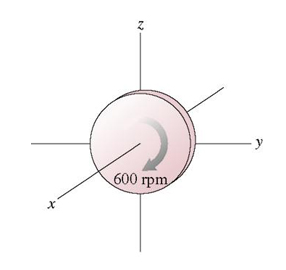# Problem: What is the magnitude of the angular momentum of the 2.60kg , 5.90-cm-diameter rotating disk in the Figure (figure is a circle with 600 rpm)?What is its direction?What is its direction?  x direction -x direction  y direction -y direction  z direction -z direction

###### FREE Expert Solution

The angular momentum of the rotating disk is expressed as:

$\overline{){\mathbf{L}}{\mathbf{=}}\frac{\mathbf{1}}{\mathbf{2}}{\mathbf{m}}{\mathbf{r}}{\mathbf{v}}}$

But v = rω

Therefore, L = (1/2)mr2ω

86% (375 ratings)###### Problem Details

What is the magnitude of the angular momentum of the 2.60kg , 5.90-cm-diameter rotating disk in the Figure (figure is a circle with 600 rpm)?What is its direction?What is its direction?  x direction
-x direction
y direction
-y direction
z direction
-z direction